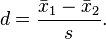## Effect of Size Calculator

 Calculate using 't values' and 'df' t value   = df   = Result: Cohen's d Effect Size (r)

 Calculate using Means and Standard Deviations Group 1 Group 2 M1= M2 = SD1= SD2=
 Result: Cohen's d Effect Size (r)

### The Effect of Size definition

In statistics, The effect size used to measure the strength of the relationship between two variables.

### Cohen's d FormulaCohen's d is defined as the difference between two means divided by a standard deviation for the data, i.e.,

Thinkcalculator.com provides you helpful and handy calculator resources.